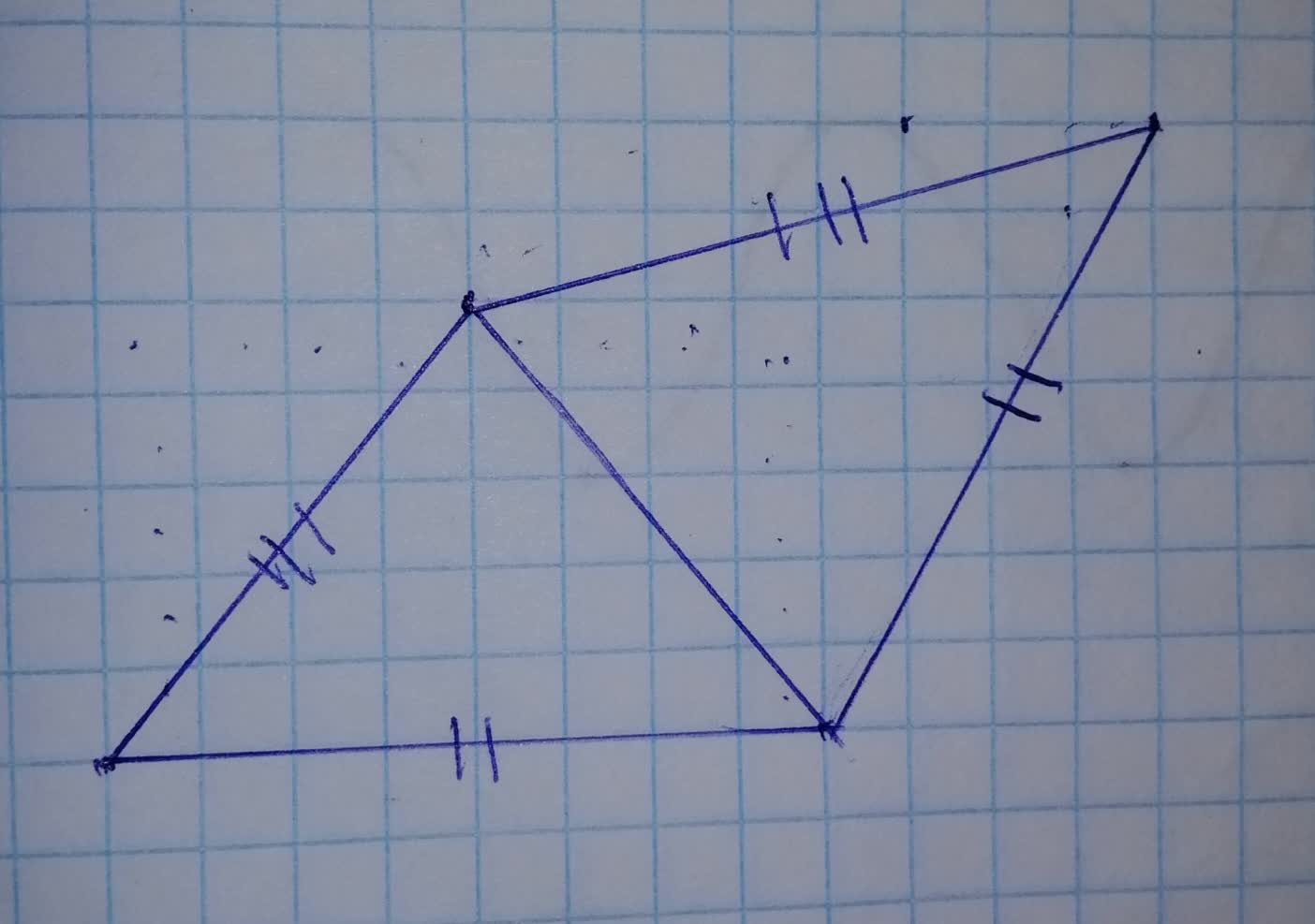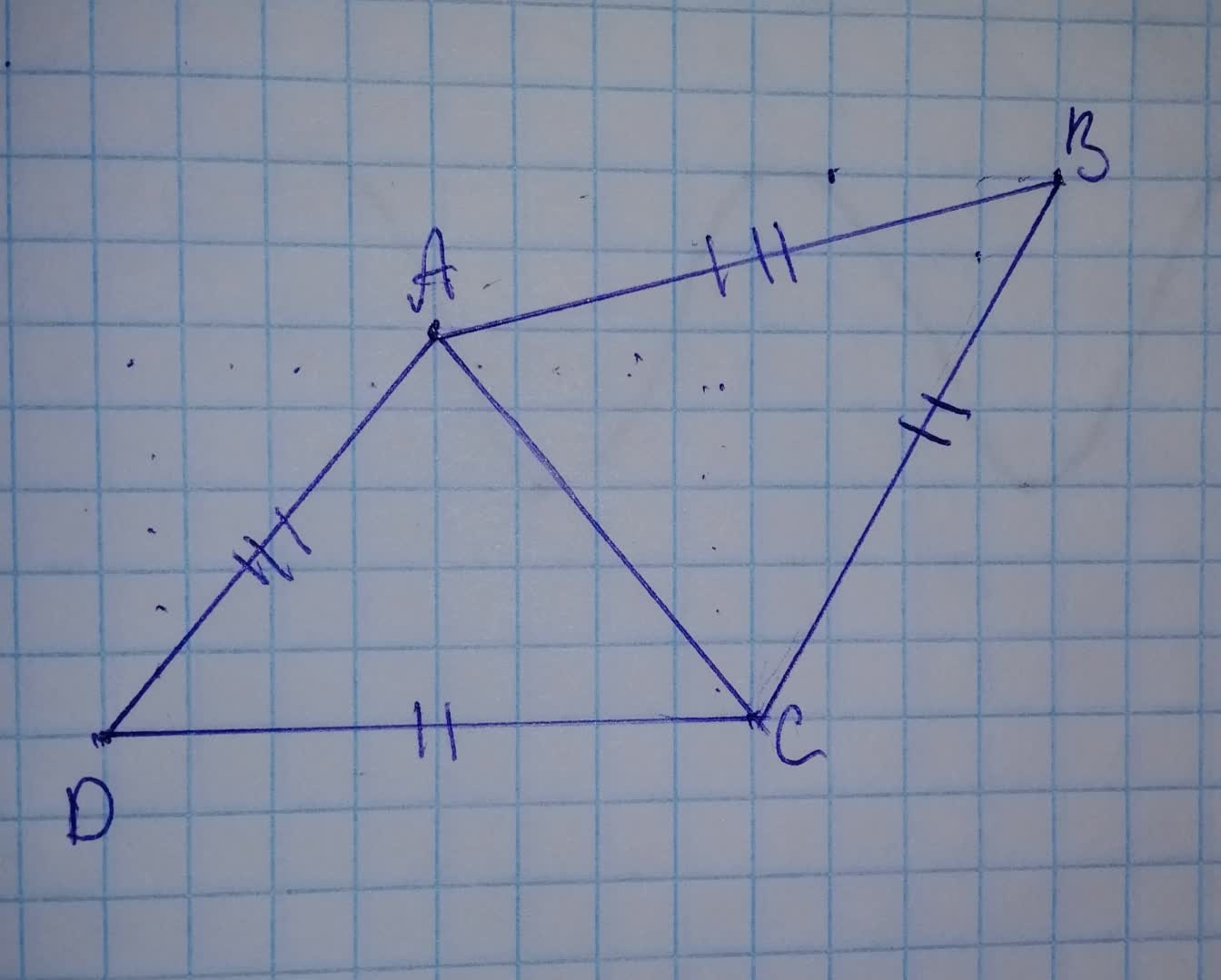# Which statement applies to these triangles: - these triangles must be congrachieverh3 2021-11-18 Answered
Which statement applies to these triangles:
- these triangles must be congruent
- these triangles might be congruent
-these triangles cannot be congruent.• Questions are typically answered in as fast as 30 minutes

### Plainmath recommends

• Get a detailed answer even on the hardest topics.
• Ask an expert for a step-by-step guidance to learn to do it yourself.Philip O'Neill
Step 1Consider $$\displaystyle\triangle{A}{D}{C}$$
and $$\displaystyle\triangle{A}{B}{C}$$
$$\displaystyle{A}{D}={A}{B}$$ (Given)
$$\displaystyle{D}{C}={B}{C}$$ (Given)
$$\displaystyle{A}{C}={A}{C}$$ (reflexive property)
Step 2
By SSS congruency
$$\displaystyle\triangle{A}{D}{C}\stackrel{\sim}{=}\triangle{A}{B}{C}$$
$$\displaystyle\therefore$$ the triangles must be congruenct.